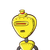# find the prime factorisation of 84 by using both division method and factor tree method​

find the prime factorisation of 84 by using both division method and factor tree method​

### 1 thought on “find the prime factorisation of 84 by using both division method and factor tree method​”

1.2,2,3,7

Step-by-step explanation:

In prime factorization we keep dividing the number (in this case 84) by a prime number until we get to 1.

84/2=42

42/2=21

21/3=7

7/7=1

Factor tree works similarly but in a different layout.

84

42               2

2       21

3        7

Hope this helped